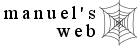nursing nursing calculators search site map

Nursing Calculators

Nursing Calculators -- On-line working calculators to solve nursing math problems with formulas and examples included.

ICU Math Quiz -- Get an idea of what nursing calculators are capable of solving.Diluting heparin for flushes

Papers

1. A case study in coercion
2. Say no to DuoDerm dressings (my pet peeve)

More

Additional and more specific dosage calculators are available at GlobalRPH.

Comprehensive ACLS ECC Algorithm (2001 guidelines)

Nursing Links --a few nursing links that may be useful or point you in the right direction.

 Apothecary/Household/Metric Equivalents

 Liquid oz     mL minim     mL 1 = 30 45 = 3 1/2 = 15 30 = 2 15 = 1 dram     mL 12 = 0.75 2 1/2 = 10 10 = 0.6 2 = 8 8 = 0.5 1 1/4 = 5 5 = 0.3 1 = 4 4 = 0.25 3 = 0.2 others 1 1/2 = 0.1 1 min (minum) = 1 gtt (drop) 1 = 0.06 1T (tablespoon) = 15mL 3/4 = 0.05 1t (teaspoon) = 5mL 1/2 = 0.03 1 ml = 1 cc Weight grain     mg grain     mg 15 = 1000 1/4 = 15 10 = 600 1/6 = 10 7 1/2 = 500 1/8 = 8 5 = 300 1/10 = 6 4 = 250 1/15 = 4 3 = 200 1/20 = 3 2 1/2 = 150 1/30 = 2 2 = 120 1/40 = 1.5 1 1/2 = 100 1/60 = 1 1 = 60 1/100 = 0.6 3/4 = 45 1/120 = 0.5 1/2 = 30 1/150 = 0.4 1/3 = 20 1/200 = 0.3 1/250 = 0.25

List of Common Drug infusions and How They Are Given

 Medication Dosage Aminophylline 0.5 - 0.7 mg/kg/hr Amrinone 5 - 15 mcg/kg/min Amiodarone 0.5 mg/min x 24hrs Bretylium 1 - 2 mg/min Diltiazem 5 - 15 mg/hr Dobutamine 2 - 20 mcg/kg/min Dopamine 1 - 20 mcg/kg/min Epinephrine 2 - 10 mcg/min Esmolol 0.05 mg/kg/min Isoproterenol 2 - 10 mcg/min Labetalol 2 - 8 mcg/min Lidocaine 30 - 50 mcg/kg/min or 2 - 4 mg/min Magnesium Sulfate 0.5 - 1 g/hr x 24 hrs Nitroglycerin 10 - 20 mcg/min Nitroprusside 0.1 - 5 mcg/kg/min Norepinephrine 0.5 - 30 mcg/min Procainamide 1-4 mg/min

### References

Many of the formulas used in the calculators were obtained from:

Munday, L. D., & Curren, A. M. (1990). Math for meds:  Dosages and solutions. (6th ed.). San Diego, CA: Wallcur Inc.

Nagelhout, J. J., & Zaglaniczy, K., L. (2001). Nurse anesthesia (2nd ed.). Philadelphia: W.B. Saunders Co.

Nagelhout, J. J., Zaglaniczy, K., L., & Haglund, V., L. (2001). Handbook of nurse anesthesia (2nd ed.). Philadelphia: W.B. Saunders Co.

Drug information obtained from:

American Heart Association. (1997). 1997-99 Handbook of emergency cardiovascular care for healthcare providers. Dallas, TX: Author.

Chohan, N., Doyle, R. M., & Johnson, P. H. (Eds.). (1999). Nursing 2000 drug handbook. Springhouse, PA: Springhouse Corporation.

Miller, R. D. (Ed.). (2000). Anesthesia. (5th ed.). Philadelphia: Churchill Livingstone.

Morgan, G. E., Jr., & Mikhail, M., S. (1996). Clinical anesthesiology. (2nd ed.). New York: Lange Medical Books/McGraw-Hill

Note: